Calculus of Finite Differences

calculus of finite differences

[′kal·kyə·ləs əv ′fī‚nīt ′dif·rən·səs]
(mathematics)
A method of interpolation that makes use of formal relations between difference operators which are, in turn, defined in terms of the values of a function on a set of equally spaced points. Also known as calculus of enlargement.

Calculus of Finite Differences

a branch of mathematics that studies functions of a discretely (discontinuously) varying argument, in contrast to differential calculus and integral calculus, where the argument is assumed to vary continuously. The expressions

Δyk ≡ Δf(xk) = f(xk+1)− f(xk)

first order differences

Δ2yk ≡ Δ2f(xk) = Δf(xk+1)− Δf(xk)

=f(xk+2) − 2f(xk+1) + f(xk+2)

second order differences

. . . . . . . . . . . . . . . . . . . .

Δnyk ≡ Δnf(xk) = Δn-1f(xk+1) − Δn-1f(xk)

are the “forward” finite differences for a sequence of values y1 = f(x1), y2 = f(x2, . . . ,yk = f(xk), . . . , of the function f(x) corresponding to the sequence x0, . . . ,xk, . . . of values of the argument; here xk = x0 + kh, with h constant and k integral.

Likewise, the “backward” finite differences Δnyk are defined by the equalities

Δnyk = ∇nyk+1

In connection with interpolation, frequent use is made of central differences δny, which are computed for odd n at the points x = xi + 1/2h and for even n at the points x = xi, by the formulas

δf(x + 1/2h) ≡ δyi+1/2 = f(xi+1) − f(xi)

δf(xi) ≡ δ2yi ≡ δyi+1/2 − δyi-1/2

. . . . . . . . . . . . . . . . . . . .

δ2m-1f(xi + 1/2h) ≡ δ2m-1yi+1/2 = δ2m-2yi+1 − δ2m

δ2mf(xi)≡δ2m-1yi+1/2 −δ2m-1yi-1/2

They are supplemented with the arithmetic means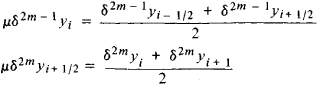for m = 1, 2, . . . and the mean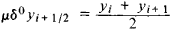for m = 0. The central differences δny are related to the finite differences Δny by the equations

δ2myi = ∆2myi-m

δ2m+1yi+1/2 = ∆2m+1yi-m

If the values of the argument do not form an arithmetic progression, that is, if xk + xk is not constant, then instead of finite differences we use divided differences, defined successively by the formulas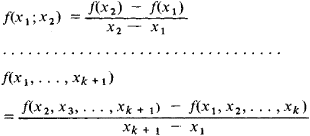The connection between finite differences and derivatives is given by the formula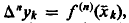, where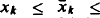. There exists a perfect analogy between the role of finite differences in the theory of functions of a discrete argument and the role of derivatives in the theory of functions of a continuous argument. Finite differences are at the core of a number of branches of numerical analysis, such as interpolation of functions, numerical differentiation and integration, and numerical methods for solving differential equations.

For example, in order to obtain an approximate solution of (an ordinary or partial) differential equation, we often replace the derivatives by the corresponding differences divided by the powers of the differences of the arguments and solve the resulting difference equation.

An important branch of the calculus of finite differences is devoted to the solution of difference equations of the form

(1)F[x, Δf(x), . . . , Δnf(x)] = 0

a problem that in many ways is similar to the solution of nth-order differential equations. Equation (1) is usually written in the form

Φ[x, f(x), f(x1), . . . , f(xn)] = 0

with differences expressed by the corresponding values of the function. A particularly simple case is that of a linear homogeneous equation with constant coefficients:

f(x + n) + a1f(x + n − 1) + . . . + anf(x) = 0

where a1, . . . , an are constants. In order to solve this equation, we must find the roots λ1, λ2, . . . , λn of its characteristic equation

λn + n-1 + . . . + an = 0

The solution of the equation is then represented in the form

f(x) = C1λ1x +C2λ2x + . . . + Cnλnx

where C1, C2, . . . , Cn are arbitrary constants (it is assumed here that no two of the numbers λ1, λ2, . . . , λn are equal).

REFERENCES

Berezin, I. S., and N. P. Zhidkov. Metody vychislenii, 3rd ed., vols. 1–2. Moscow, 1966.
Gel’fond, A. O. Ischislenie konechnykh raznostei, 3rd ed. Moscow, 1967. edited by

N. S. BAKHVALOV

Site: Follow: Share:
Open / Close